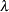MODEL Statement
MODEL dependent = <regressors> </ options> ;

The MODEL statement specifies the dependent variable and independent covariates (regressors) for the regression model. If you specify no regressors, PROC TCOUNTREG fits a model that contains only an intercept. The dependent count variable should take on only nonnegative integer values in the input data set. PROC TCOUNTREG rounds any positive noninteger count values to the nearest integer. PROC TCOUNTREG ignores any observations with a negative count.

Only one MODEL statement can be specified. The following options can be used in the MODEL statement after a slash (/).

DIST=value

specifies a type of model to be analyzed. If you specify this option in both the MODEL statement and the PROC TCOUNTREG statement, then only the value in the MODEL statement is used. The following model types are supported:

POISSON | P

specifies a Poisson regression model.

NEGBIN(P=1)

specifies a negative binomial regression model with a linear variance function.

NEGBIN(P=2) | NEGBIN

specifies a negative binomial regression model with a quadratic variance function.

ZIPOISSON | ZIP

specifies a zero-inflated Poisson regression. The ZEROMODEL statement must be specified when this model type is specified.

ZINEGBIN | ZINB

specifies a zero-inflated negative binomial regression. The ZEROMODEL statement must be specified when this model type is specified.

ERRORCOMP=value

specifies a type of conditional panel model to be analyzed. The following model types are supported:

FIXED

specifies a fixed-effect error component regression model.

RANDOM

specifies a random-effect error component regression model.

NOINT

suppresses the intercept parameter.

OFFSET=variable

specifies a variable in the input data set to be used as an offset variable. The offset variable appears as a covariate in the model with its parameter restricted to 1. The offset variable cannot be the response variable, the zero-inflation offset variable (if any), or one of the explanatory variables. The "Model Fit Summary" table gives the name of the data set variable used as the offset variable; it is labeled as "Offset."

SELECT=(INFO(option) | PEN(option))

specifies variable selection.

SELECT= INFO specifies that the variable selection method is based on an information criterion. For more information, see the section Variable Selection Using an Information Criterion. The following options are available for SELECT=INFO:
DIRECTION=FORWARD | BACKWARD

specifies the searching algorithm used in the variable selection method. The default is FORWARD.

CRITER=AIC | SBC

specifies the information criterion used for the variable selection. The default is SBC.

MAXSTEPS=value

specifies the maximum number of steps allowed in the search algorithm. The default is infinite; that is, the algorithm does not stop until the stopping criterion is satified.

LSTOP=value

specifies the stopping criterion. value represents the percentage of decrease or increase in the AIC or SBC that is required for the algorithm to proceed; it must be a positive number less than 1. The default is zero.

SELECT=PEN specifies that the variable selection method is penalized likelihood. For more information, see the section Variable Selection Using Penalized Likelihood. The following options are available for SELECT=PEN:
LLASTEPS=value

specifies the maximum number of iterations in the algorithm of local linear approximations. The default is 5.

GCVLENGTH=value

specifies the number of different values that is used for the generalized cross-validation (GCV) tuning parameter. The value corresponds toin the computations described in the section Variable Selection Using Penalized Likelihood. The default value is 20.

GCV

specifies that the generalized cross-validation (GCV) approach be used. For more information, see the section The GCV Approach.

GCV1

specifies that the GCV1 approach be used. For more information, see the section The GCV1 Approach. GCV1 is the default.

Printing Options

CORRB

prints the correlation matrix of the parameter estimates. The CORRB option can also be specified in the PROC TCOUNTREG statement.

COVB

prints the covariance matrix of the parameter estimates. The COVB can also be specified in the PROC TCOUNTREG statement.

ITPRINT

prints the objective function and parameter estimates at each iteration. The objective function is the negative log-likelihood function. The ITPRINT option can also be specified in the PROC TCOUNTREG statement.

PRINTALL

requests all printing options. The PRINTALL option can also be specified in the PROC TCOUNTREG statement.

Note: This procedure is experimental.